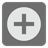Count Consecutive Summers
Simple
English RU

Positive integers can be expressed as sums of consecutive positive integers in various ways. For example, 42 can be expressed as such a sum in four different ways:(a) 3+4+5+6+7+8+9, (b) 9+10+11+12, (c) 13+14+15 and (d) 42. As the last solution (d) shows, any positive integer can always be trivially expressed as a singleton sum that consists of that integer alone.

Compute how many different ways it can be expressed as a sum of consecutive positive integers.

Input: Int.

Output: Int.

Example:

```count_consecutive_summers(42) == 4
count_consecutive_summers(99) == 6
```

Precondition: Input is always a positive integer.

The mission was taken from Python CCPS 109 Fall 2018. It’s being taught for Ryerson Chang School of Continuing Education by Ilkka Kokkarinen